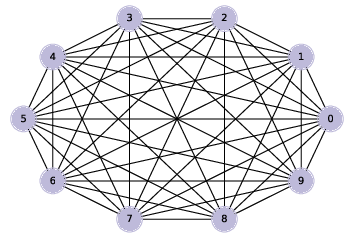# 4.5. Generating Graphs¶Figure 4.3: A complete graph with 10 nodes.¶

We’ll start by generating a complete graph, which is a graph where every node is connected to every other.

Here’s a generator function that takes a list of nodes and enumerates all distinct pairs. If you are not familiar with generator functions.

```def all_pairs(nodes):
for i, u in enumerate(nodes):
for j, v in enumerate(nodes):
if i>j:
yield u, v
```

We can use `all_pairs` to construct a complete graph:

```def make_complete_graph(n):
G = nx.Graph()
nodes = range(n)
return G
```

`make_complete_graph` takes the number of nodes, `n`, and returns a new `Graph` with `n` nodes and edges between all pairs of nodes.

The following code makes a complete graph with 10 nodes and draws it:

```complete = make_complete_graph(10)
nx.draw_circular(complete,
node_color=COLORS,
node_size=1000,
with_labels=True)
```

Figure 4.3 shows the result. Soon we will modify this code to generate ER graphs, but first we’ll develop functions to check whether a graph is connected.

You have attempted of activities on this page